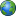Home | News Update | English Language | Mathematics | Physics | Biology | Geography | Forum | About Us
Fast & Easy Contact??08080092411 ||Coming soon..
Mathematics_pictures
think
lenght
learn
Maths Literacy - Ratios and Percentages
Sequences and series
estimating surds
factorisation
logarithms
rounding off
simplification of fractions
word problems
standard formA quadratic equation is an equation where the exponent of the variable is at most 2.

The quadratic must first be factored, because it is only when you MULTIPLY and get zero that you can say anything about the factors and solutions. You can’t conclude anything about the individual terms of the unfactored quadratic (like the 5 x or the 6), because you can add lots of stuff that totals zero.

So the first thing I have to do is factor: x2 + 5x + 6 = (x + 2)(x + 3)

Set this equal to zero: (x + 2)(x + 3) = 0

Solve each factor: x + 2 = 0 or x + 3 = 0 x = –2 or x = – 3

The solution to x2 + 5x + 6 = 0 is x = –3, –2

Checking x = –3 and x = –2 in x2 + 5x + 6 = 0:

[–3]2 + 5[–3] + 6 ?=? 0

9 – 15 + 6 ?=? 0

9 + 6 – 15 ?=? 0

15 – 15 ?=?

0 0 = 0

[–2]2 + 5[–2] + 6 ?=? 0

4 – 10 + 6 ?=? 0

4 + 6 – 10 ?=? 0

10 – 10 ?=? 0

0 = 0

Exercise 2:

Quadratic Equations This equation is not in “(quadratic) equals (zero)” form, so I can’t try to solve it yet. The first thing I need to do is get all the terms over on one side, with zero on the other side. Only then can I factor and solve:

x2 – 3 = 2x

x2 – 2x – 3 = 0

(x – 3)(x + 1) = 0

x – 3 = 0 or x + 1 = 0

x = 3 or x = –1

Then the solution to x2 – 3 = 2x is x = –1, 3Share Your Story With The World Part of Uzomedia site) site owned and manage by Uzomedia..Polly po-cket